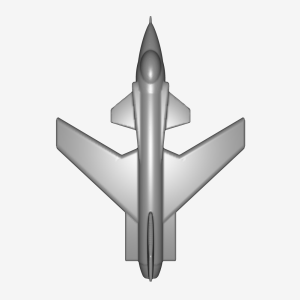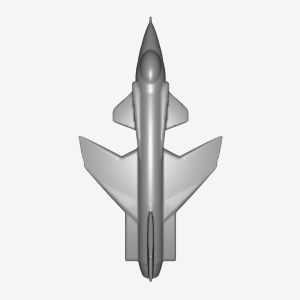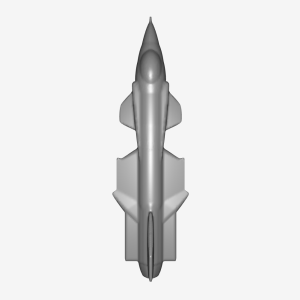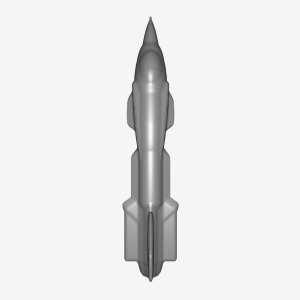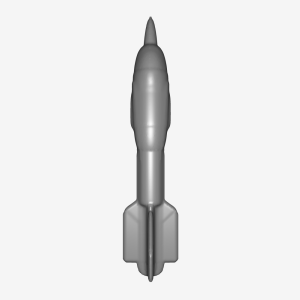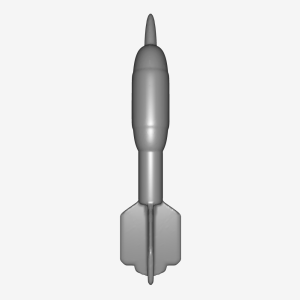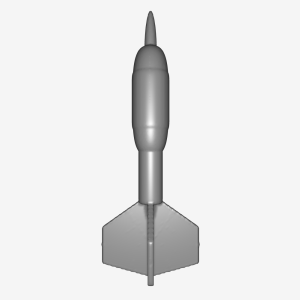3D Scan Conversion of CSG Models Into Distance Volumes

David Breen *  Sean Mauch *  Ross Whitaker **

* Computer Graphics Lab, Caltech    ** School of Computing, U. of Utah

A distance volume is a volume dataset where the value stored at each voxel is the shortest distance to the surface of the object being represented by the volume. Distance volumes are a useful representation in a number of computer graphics applications. We have developed a technique for generating a distance volume from one type of geometric model, a Constructive Solid Geometry (CSG) model consisting of superellipsoid primitives. (See Breen, Mauch & Whitaker 1998, 1999, 2000). The distance volume is generated in a two step process. The first step calculates the shortest distance to the CSG model at a set of points within a narrow band around the evaluated surface. Additionally, a second set of points, labeled the zero set, which lies on the CSG model's surface are computed. A point in the zero set is associated with each point in the narrow band. Once the narrow band and zero set are calculated a fast marching method is employed to propagate the shortest distance and closest point information out to the remaining voxels in the volume. Our technique has been used to scan convert a number of CSG models, producing distance volumes which have been utilized in a variety of computer graphics applications, e.g. CSG surface evaluation, offset surface generation, and 3-D model morphing.

Generating the Narrow Band and Zero Set

The narrow band and zero set needed for the fast marching method are generated with a modified version of the Constructive Cubes algorithm (See Breen 1991). For each voxel, the Constructive Cubes algorithm traverses the CSG model's acyclic graph, evaluates each primitive's inside-outside function at the voxel location, and combines sub-component values at each non-leaf node of the graph to produce a value which represents the inside-outside function for the complete model at a particular point. The Constructive Cubes algorithm has been modified in two ways to generate the data needed for the fast marching algorithm. First, the step which evaluates the inside-outside function for each superellipsoid at a particular point has been replaced by a technique for calculating the closest point and shortest distance to the superellipsoid from an arbitrary point. The second modification involves formulating new shortest distance combination rules which are applied at each non-leaf node of the CSG graph. These are formulated in a way to produce the closest point and shortest distance to the entire CSG model from a point near the model surface.

Fast Marching Method

To calculate the closest points to a surface on a regular grid, we utilize an algorithm similar to Sethian's fast marching method, but instead of using a finite difference scheme to compute distance, we use a heuristic algorithm to propagate closest points information. Instead of specifying the distance for the points in the narrow band as an initial condition, we specify the closest points to the surface. In one step of the closest points method:
• The point gp with the smallest distance is removed from the narrow band and it's value is frozen.
• Points are added to the narrow band to maintain unit thickness.
• The closest points of the neighbors with larger distances than gp are recomputed using the closest point information from gp.

Research Results

CSG Model Surface Evaluation

The figure on the left is a CSG model evaluated with the original Constructive Cubes algorithm. The image on the right demonstrates that evaluating a similar CSG model using distance volumes produces superior results.

The following two figures present a dart CSG model and an X-29 jet fighter CSG model. Their surfaces have been evaluated by first performing 3D scan conversion at a resolution of 96x192x240. The iso-surface at value zero is then extracted from the distance volume using the Marching Cubes algorithm.

Offset Surface Generation

Extracting an iso-surface at a value other than zero produces offset surfaces of the CSG model. The following images present offset surfaces from the X-29 model at values .15, .25, .5, and .8.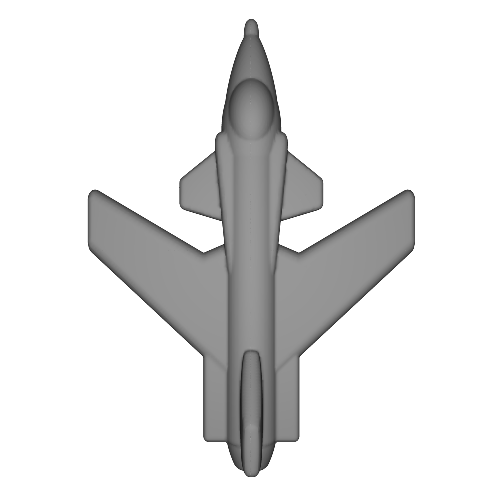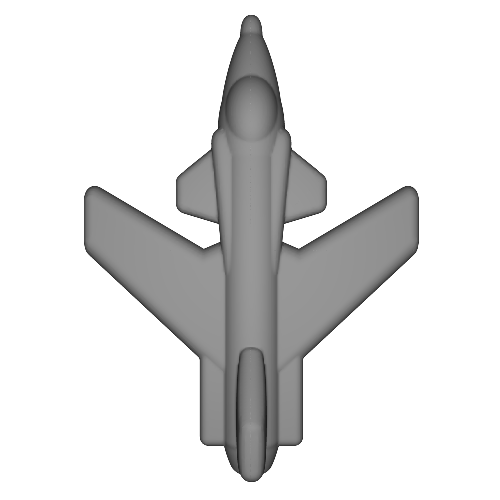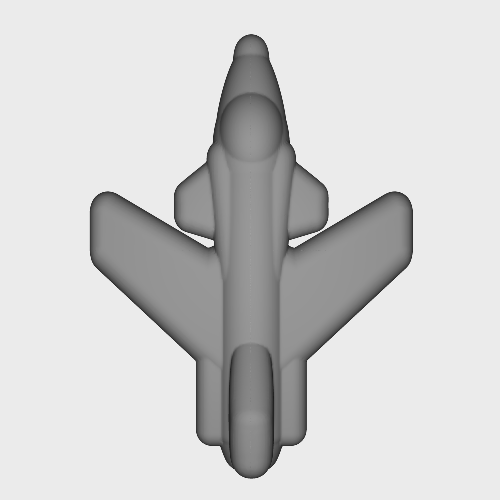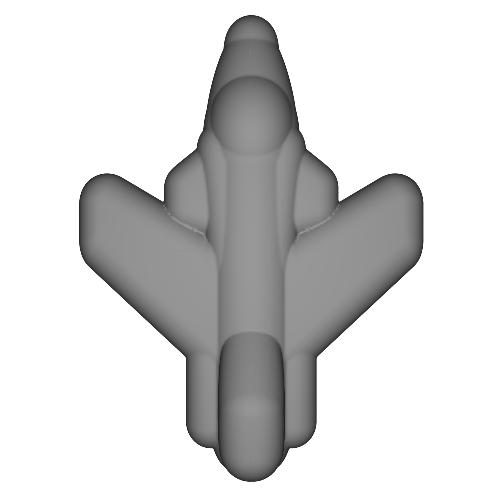3D Model Morphing

Distance volumes provide the input representation for our 3D model morphing work using Level Sets. The following are frames from an animation of a X-29 jet morphing into a dart.